Question

Explain your answer in depth - show formulas and all relevant stuff The current market price of a security is \$50, the security's expected return is 15%, the riskless rate of interest is 2%, and...

Explain your answer in depth - show formulas and all relevant stuff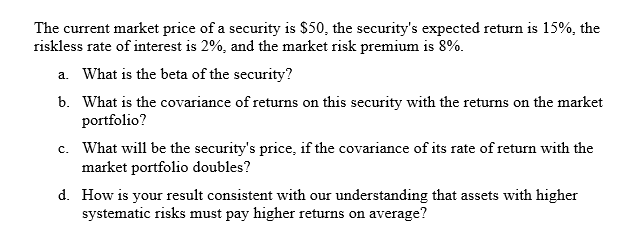The current market price of a security is \$50, the security's expected return is 15%, the riskless rate of interest is 2%, and the market risk premium is 8% What is the beta of the security? What is the covariance of returns on this portfolio? What will be the security's price, if the covariance of its rate of return with the market portfolio doubles? How is your result consistent with our understanding that assets with higher systematic risks must pay higher returns on average? a. b. security with the re turns on the market c. d.

Current Market price of the security = \$50

Security Expected return = E[Ri] = 15%

Risk-free rate = Rf = 2%

Market Risk premium is the difference between return on market portfolio and the risk free rate.

Market Risk Premium = RM-Rf = 8%

a. We will use the CAPM equation

CAPM Equation

E[Ri] = Rf + βi*(RM - Rf)

15% = 2% + βi *(8%)

βi = 17%/8% = 2.125

Therefore, Beta of the stock = 2.125

b. Below is the formula for beta of a stock i

βi = Cov(i, M)/σM2

where Cov(i,M) is the covariance of return on security i with the return on the market portfolio M.

Cov(i, M) = βi* σM2

Therefore, the covariance between security return and the market is the product of the beta of the security and market variance.

c. It is given that the covariance of its rate of return with the market portfolio doubles. Now, since the variance of the return on market portfolio (σM2) doesn’t change and Cov(i, M) = βi* σM2, Hence, the beta of the stock will also get double. (From the above answer)

Therefore, βi,new = 2*2.125 = 4.25

Using CAPM Equation

E[Ri] = Rf + βi,new*(RM - Rf) = 2% + 4.25*(8%) = 36%

New Expected return = 36%

Therefore, new security’s price = (1+36%)*50 = \$60

d. We know that beta is a measure of Stock’s volatility with respect to the market or systematic risk. As the beta doubled from 2.125 to 4.25 i.e., the systematic risk increased, we can see that the return increased from 15% to 36%. Hence, the result is consistent with the understanding that assets with higher systematic risk must pay higher return on average.

Earn Coins

Coins can be redeemed for fabulous gifts.

Similar Homework Help Questions
• The market price of a security is \$50. Its expected rate of return is 14%. The...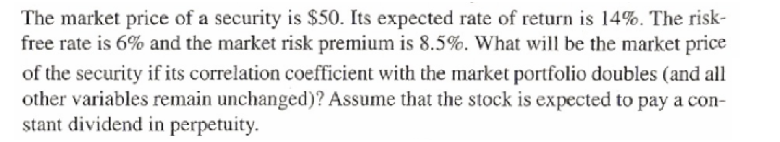The market price of a security is \$50. Its expected rate of return is 14%. The risk- free rate is 6% and the market risk premium is 8.5%. What will be the market price of the security if its correlation coefficient with the market portfolio doubles and all other variables remain unchanged)? Assume that the stock is expected to pay a con- stant dividend in perpetuity.

• E A B The market price of a security is \$40. Its expected rate of return...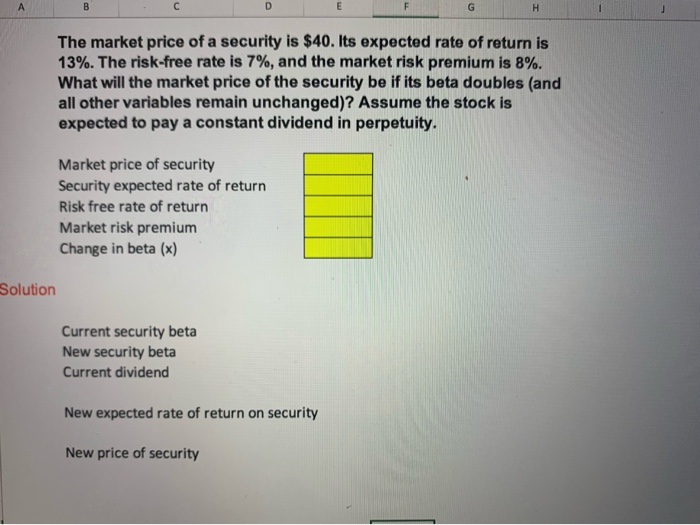E A B The market price of a security is \$40. Its expected rate of return is 13%. The risk-free rate is 7%, and the market risk premium is 8% . What will the market price of the security be if its beta doubles (and all other variables remain unchanged)? Assume the stock is expected to pay a constant dividend in perpetuity. Market price of security Security expected rate of return Risk free rate of return Market risk premium Change...

• The market price of a security is \$50. Its expected rate of return is 14%. The risk- free rate is 6% and the market ris...The market price of a security is \$50. Its expected rate of return is 14%. The risk- free rate is 6% and the market risk premium is 8.5%. What will be the market price of the security if its correlation coefficient with the market portfolio doubles (and all other variables remain unchanged)? Assume that the stock is expected to pay a con stant dividend in perpetuity

• The market price of a security is \$64. Its expected rate of return is 13%. The...

The market price of a security is \$64. Its expected rate of return is 13%. The risk-free rate is 6%, and the market risk premium is 9%. What will the market price of the security be if its beta doubles (and all other variables remain unchanged)? Assume the stock is expected to pay a constant dividend in perpetuity.

• The market price of a security is \$26. Its expected rate of return is 13%. The...

The market price of a security is \$26. Its expected rate of return is 13%. The risk-free rate is 5% and the market risk premium is 7.0%. What will be the market price of the security if its correlation coefficient with the market portfolio doubles (and all other variables remain unchanged)? Assume that the stock is expected to pay a constant dividend in perpetuity. (Do not round intermediate calculations. Round your answer to 2 decimal places.)

• Ch 02: Assignment - Risk and Return: Part 1 Term Answer Risk A Expected rate of...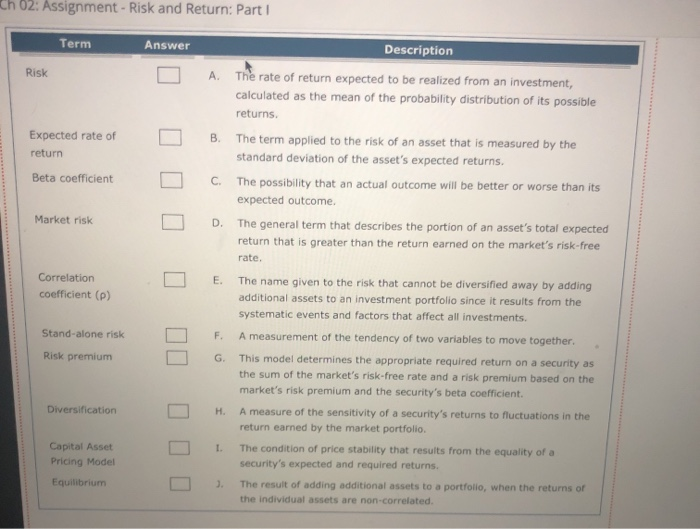Ch 02: Assignment - Risk and Return: Part 1 Term Answer Risk A Expected rate of return B Description The rate of return expected to be realized from an investment, calculated as the mean of the probability distribution of its possible returns. The term applied to the risk of an asset that is measured by the standard deviation of the asset's expected returns. The possibility that an actual outcome will be better or worse than its expected outcome The general...

• Security ABC has a price of \$35 and a beta of 1.5. The risk-free rate is...

Security ABC has a price of \$35 and a beta of 1.5. The risk-free rate is 5% and the market risk premium is 6%. a)Explain the terms beta and market risk premium. b)What is the market portfolio? c)According to the CAPM, what return do investors expect on the security? d)Investors expect the security not to pay any dividend next year. e)At what price do investors expect the security to trade next year? f)At what price do investors expect the security...

• 5. Assume CAPM holds: The market price of a security is \$50. Its expected rate of...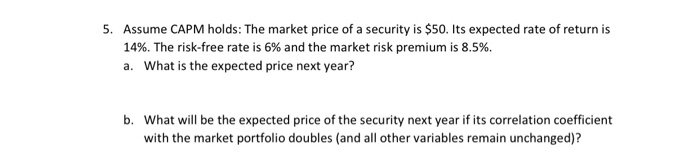5. Assume CAPM holds: The market price of a security is \$50. Its expected rate of return is 14%. The risk-free rate is 6% and the market risk premium is 8.5%. a. What is the expected price next year? b. What will be the expected price of the security next year if its correlation coefficient with the market portfolio doubles (and all other variables remain unchanged)?

• Security ABC has a price of \$35 and a beta of 1.5. The risk-free rate is...

Security ABC has a price of \$35 and a beta of 1.5. The risk-free rate is 5% and the market risk premium is 6%. According to the CAPM, what return do investors expect on the security? Investors expect the security not to pay any dividend next year. At what price do investors expect the security to trade next year? At what price do investors expect the security to trade next year, if the expected dividend next year is \$2 instead...

• Choose the correct answer and explain briefly 8. What is the expected return of a zero-beta...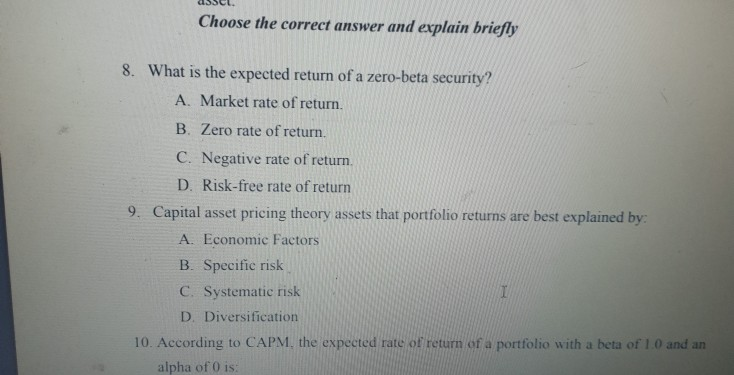Choose the correct answer and explain briefly 8. What is the expected return of a zero-beta security? A. Market rate of return. B Zero rate of return. C. Negative rate of return. D. Risk-free rate of return 9. Capital asset pricing theory assets that portfolio returns are best explained by: A. Economic Factors B. Specific risk C. Systematic risk I D. Diversification 10. According to CAPM, the expected rate of return of a portfolio with a beta of 1.0 and...